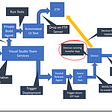# How to create sounds with specific characteristics?

How can I create sounds with MATLAB with the following characteristics:

Sound 1:

`pitch: 3 sinusoidal partials of 500, 1000  and 1500 Hz duration: 100 ms (including 5 ms rise and fall times) amplitude: 80 dB`

Sound 2:

`like Sound 1, but with a cutting out of 10 ms (5 ms fall / rise included) from the middle of the sound`

This is my third try

`%%configure signal settings duration = 0.1;                % duration in seconds amplitude = 0.8;               % amplitude f1 = 500;                      % frequency in Hertz f2 = 1000; f3 = 1500; phi = 2*pi*0.5;                % phase offset, e.g.: 2*pi*0.25 = 1/4 cycle %%configure output settings fs = 44100;                    % sampling rate T = 1/fs;                      % sampling period t = 0:T:duration;              % time vector %%create the signal omega1 = 2*pi*f1;              % angular frequency in radians omega2 = 2*pi*f2; omega3 = 2*pi*f3; partial1 = cos(omega1*t + phi)*amplitude;      % sinusoidal partial 1 partial2 = cos(omega2*t + phi)*amplitude;      % sinusoidal partial 2 partial3 = cos(omega3*t + phi)*amplitude;      % sinusoidal partial 3 signal = (partial1 + partial2 + partial3)/3; %%plot the signal plot(t, signal); xlabel('Time (seconds)'); ylabel('Amplitude'); title('Complex Signal'); %%play the signal sound(signal, fs); %%save signal as stereo wave file stereo_signal = [x1; x1]'; wavwrite(stereo_signal, fs, 'test.wav');`

I am wondering how to adjust the amplitude and to implement the rise and fall.

NOTE:-

Matlabsolutions.com provide latest MatLab Homework Help,MatLab Assignment Help for students, engineers and researchers in Multiple Branches like ECE, EEE, CSE, Mechanical, Civil with 100% output.Matlab Code for B.E, B.Tech,M.E,M.Tech, Ph.D. Scholars with 100% privacy guaranteed. Get MATLAB projects with source code for your learning and research.

A window can be anything going from zero to unity. In this case it should reach unity after 5ms from the start. You should know what kind of performance you are looking for, so you can determine what response your window should have.

Often I start with 1/4 of a sine period, since it has the nice properties to start with a ramp and that its derivative is continuous when it meets unity. For instance for time array t with begin time b and end time e of the window;

`w = ((1+sin(pi*(t-b)/2/e))/2.*(t>b-e)-1 ) .*(t<=b+e)+ 1;`

This equals 0 for t<b, then increases and equals 1 for t>e. Also note that Matlab

--

--

--

## More from Technical Source

Simple! That is me, a simple person. I am passionate about knowledge and reading. That’s why I have decided to write and share a bit of my life and thoughts to.

Love podcasts or audiobooks? Learn on the go with our new app.

## Elixir + Kubernetes = 💜 (Part 1)## Micronaut application in heroku## MyRoad2Code #1: Leaving my Job and Learning to Code## Dart Learning [Part 1]## Infrastructure as Code on AWS in a familiar language — the right way with InGraph## DevOps for IoT ( Part 2) — Installing an app from within an app## The FANATEC Formula V2 Steering Wheel Review (Compared with the Clubsport F1 Esports wheel)## Technical Source

Simple! That is me, a simple person. I am passionate about knowledge and reading. That’s why I have decided to write and share a bit of my life and thoughts to.

## How can you plot lines of latitude and longitude on a globe without using the mapping toolbox ?## Neural Network, Recurrent Neural Network, Backpropagation Algorithm, Vanishing Gradient Problem.